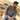# How to use objects in JavaScriptTaimoor Sattar

In JavaScript, we have 5 types of primitive data types:

• string
• number
• boolean
• null
• undefined

The primitive data type in javascript has a single value, but the object has multiple values and it can also define the function inside of the object. Data in an object defined as a key/value pair. We can define an object in javascript as below:

``````var person = {
firstName: "Taimoor",
lastName: "Sattar"
}``````

In javascript, to access the keys of object using the below command:

``````Object.keys(person)

// output
// ["firstName", "lastName"]``````

In javascript, to access the values of object using the below command:

``````Object.values(person)

// output
// ["Taimoor", "Sattar"]``````

In javascript, to access the keys and values of object using the below command:

``````Object.entries(person)

// output
// [["firstName", "Taimoor"], ["lastName", "Sattar"]]``````

We can access each value of an object using the below command in Javascript

``````// 1.
person.firstName

// 2.
person["firstName"]``````

Now, let add more data inside of person object. Change the person object as below:

``````var person = {
firstName: "Taimoor",
lastName: "Sattar",
age: 23,
mata: {
color: "brown",
eyeColor: "brown"
},
fullName: function() {
return `\${this.firstName} \${this.lastName}`;
}
};``````

In order to get a person full name, we can use the below command in javascript

``person.fullName()``

`fullName()` is the function in the person object that returns the full name of person. We use `this` in the function to get the reference for a person object.

We can also define an object with an object. As in the person object, we have a meta object and have two key values in it. To get the color value inside of meta-object, we use the below command in javascript.

``let color = person.mata.color``

Now, let suppose if to access the meta value e.g. height in person object; it will return the undefined as it does not exist. To avoid the undefined return value, you can type the command as below:

``let height = person?.mata?.height ?? ""``

If the height exists, it returns that value else it will return an empty string.

Last, to delete the specific property in person object e.g. age, we can use the below command:

``delete person.age;``